# Coffee

In stock are three kinds of branded coffee prices:

I. kind......205 Kc/kg
II. kind......274 Kc/kg
III. kind.....168 Kc/kg

Mixing these three species in the ratio 8:5:6 create a mixture. What will be the price of 100 grams of this mixture?

Result

x =  21.147 Kc

#### Solution:

$m_{1}=100 \ g \rightarrow kg=100 / 1000 \ kg=0.1 \ kg \ \\ \ \\ r=\dfrac{ 8 \cdot \ 205+5 \cdot \ 274+6 \cdot \ 168 }{ 8+5+6 }=\dfrac{ 4018 }{ 19 } \doteq 211.4737 \ \text{Kc/kg} \ \\ \ \\ x=r \cdot \ m_{1}=211.4737 \cdot \ 0.1 \doteq \dfrac{ 2009 }{ 95 } \doteq 21.1474 \doteq 21.147 \ \text{Kc}$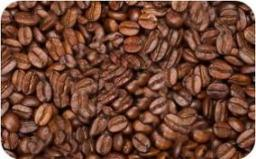Our examples were largely sent or created by pupils and students themselves. Therefore, we would be pleased if you could send us any errors you found, spelling mistakes, or rephasing the example. Thank you!

Leave us a comment of this math problem and its solution (i.e. if it is still somewhat unclear...):Be the first to comment!Tips to related online calculators
Looking for a statistical calculator?
Do you have a linear equation or system of equations and looking for its solution? Or do you have quadratic equation?

## Next similar math problems:

1. Job applicantsJob applicants: three-fourths of applicants had experience for a position. The number that did not have prior experience was 36. How many people applied for the job?
2. A mapA map with a scale of 1: 5,000 shows a rectangular field with an area of 18 ha. The length of the field is three times its width. The area of the field on the map is 72 cm square. What is the actual length and width of the field?
3. Ducats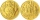The king divided the ducats to his three sons in a ratio of 2: 5: 4. How many ducats the king divided them if the youngest received 260 ducats, which was the least of all sons.
4. Using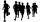Using one of the following forms x+p=q or px=q write an to represent these problems using x as the unknown variable Larry ran 7 more miles than Barry in a month, if Larry ran 20 miles how many did Barry run? please help its due tomorrow
5. What time is it?What time is it, when there is 4 times less time left until midnight than the time that has elapsed since noon? What time is it, when one-fifth of the hours that have passed since midnight is equal to one-third of the hours that are missing by 12 o'clock?
6. The farmerThe farmer calculated that the supply of fodder for his 20 cows was enough for 60 days. He decided to sell 2 cows and a third of the feed. How long will the feed for the rest of the peasant's herd last?
7. LibraryNew books were purchased for the library. Five-eighths were professional books, one-fifth were encyclopedias, and 231 books were dictionaries. How many professional books were there?
8. Large familyThe average age of all family members (children, mother, father, grandmother, grandfather) is 29 years. The average age of parents is 40 years, grandparents 66 years and all children are 5 years. How many children are there in this family?
9. A bucketA bucket has 4 liters of water in it when it is 2/5 full. How much can it hold?
10. The orchard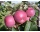4 temporary workers harvested the orchard in 9 days. How many temporary workers we need for six days?
11. TroopsThere are two leaders in the tourist group, with an average age of 30 years and several children with an average age of 10 years. The total age of the group is 12 years. How many children are in the group?
12. Nádoba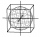Nádoba tvaru kostky je naplněna vodou do poloviny své výšky. Pokud dolijeme 20 l vody, bude nádoba naplněna do tří čtvrtin své výšky. Jaký je objem celé nádoby?
13. Equal temperature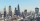The temperatures of the two cities were measured at the same time. The temperature in city A was 60 degrees And rose at a constant rate of 2 degrees per hour. The temperature in city B was 40° and rose at a constant rate of 10° per hour Enter the tim
14. Christmas or EasterPlease calculate this example by the Venn equation. They asked 73 students whether they like Christmas or Easter. 34 of them like one of the holidays. 39 loves Easter. There are twice as many students who wish both holidays than those who only love Ea
15. Tributaries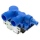The pool can be filled with two different tributaries. The first inflow would fill the pool in 18 hours, both in 6 hours. How many hours would the pool filled with a second inflow?
16. Two machinesThe first machine can produce parts in 24 hours, the second in 16 hours. The first started working in the morning at 6:00 pm. Second at 10:00 o'clock. At what hour were all parts made?
17. RectanglesVladimir likes to draw rectangles. Yesterday he created all rectangles that had sides in centimeters and a circumference of 18 cm. How many rectangles of different dimensions have been drawn?